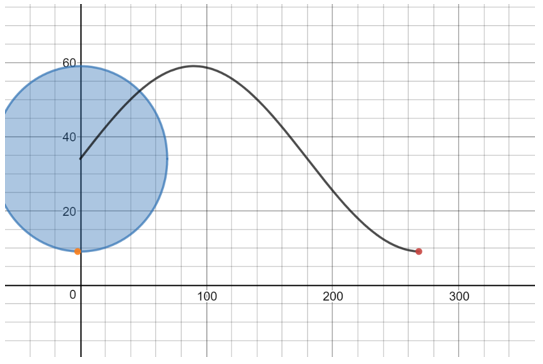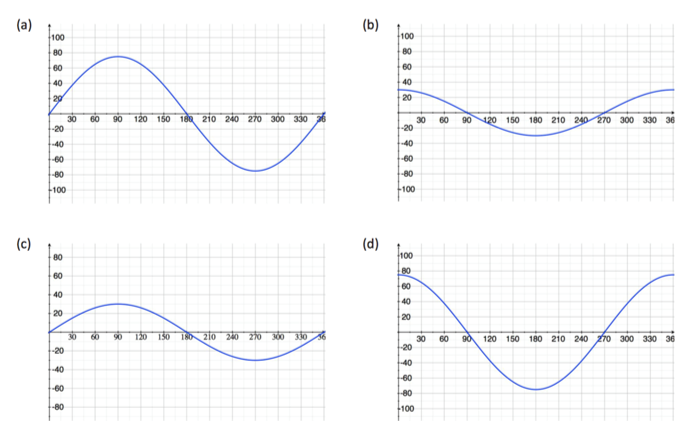# Unit Circle and Trigonometric Functions

## Objective

Sketch cosine graphs to model contextual situations and identify features of cosine graphs.

## Common Core Standards

### Core Standards

?

• F.IF.C.7.E — Graph exponential and logarithmic functions, showing intercepts and end behavior, and trigonometric functions, showing period, midline, and amplitude.

• F.TF.B.5 — Choose trigonometric functions to model periodic phenomena with specified amplitude, frequency, and midline. Modeling is best interpreted not as a collection of isolated topics but in relation to other standards. Making mathematical models is a Standard for Mathematical Practice, and specific modeling standards appear throughout the high school standards indicated by a star symbol (★). The star symbol sometimes appears on the heading for a group of standards; in that case, it should be understood to apply to all standards in that group.

?

• G.SRT.C.6

## Criteria for Success

?

1. Identify appropriate situations for a sinusoidal model.
2. Connect cosine functions with the height of a rotating object over time.
3. Identify midline, phase shift, period, and amplitude of cosine functions.
4. Sketch a cosine graph to model a contextual situation.
5. Identify differences between sine and cosine functions.

## Anchor Problems

?

### Problem 1Graphed above is a sine function, which measures the height of the point. What if, instead, a function measured the horizontal distance from the y-axis? Sketch what that function might look like.

### Problem 2

Show the first video of Ferris Wheel by Dan Meyer.

What would the graph look like if you graphed horizontal distance rather than vertical distance? At what point on the cosine graph will riders get on and off?

#### References

101Questions Ferris Wheel

Ferris Wheel by Dan Meyer is made available on 101Questions under the CC BY 3.0 license. Accessed Feb. 26, 2018, 10:38 a.m..

## Problem Set

?

The following resources include problems and activities aligned to the objective of the lesson that can be used to create your own problem set.

• Include problems connecting graphs and contextual situations.
• Include problems finding periods of graphs and extrapolating to emphasize periodic behavior.
• Include problems that measure the x-axis both in time and in degrees.

?

Zeke Memorial Park has two different-sized Ferris wheels, one with a radius of 75 feet and one with a radius of 30 feet. For either wheel, riders board at the 3 o’clock position. Distances are measured from the center of the wheel, and rotations are measured in degrees.

For each of the letters (a)–(d), answer the following questions: